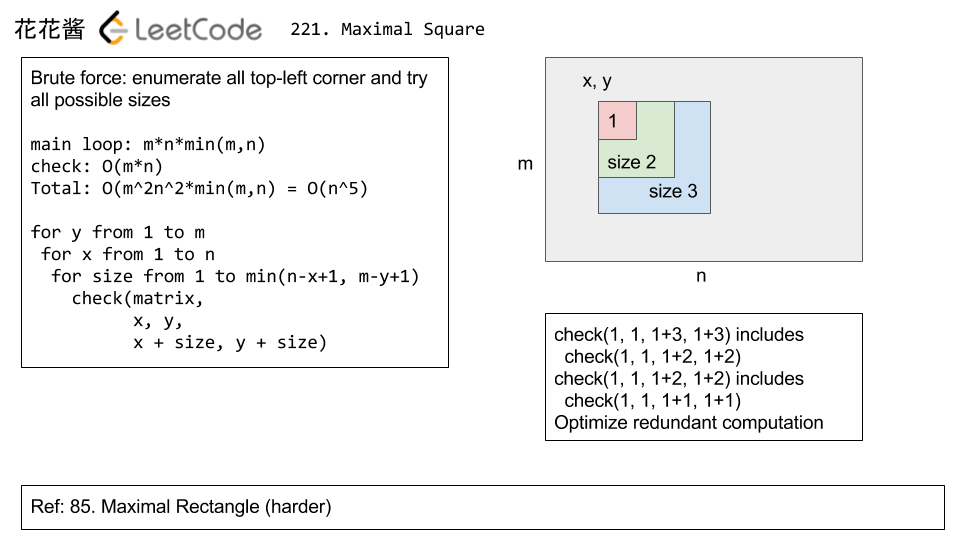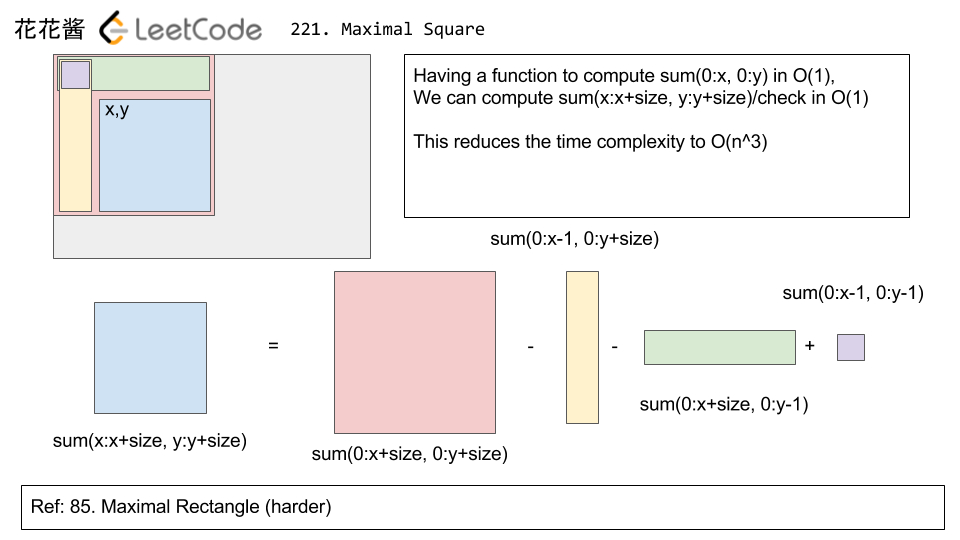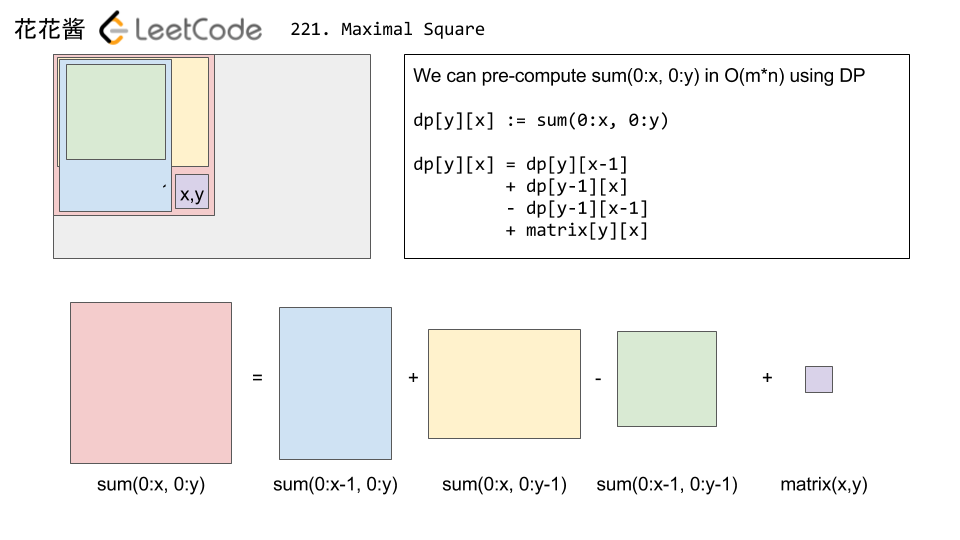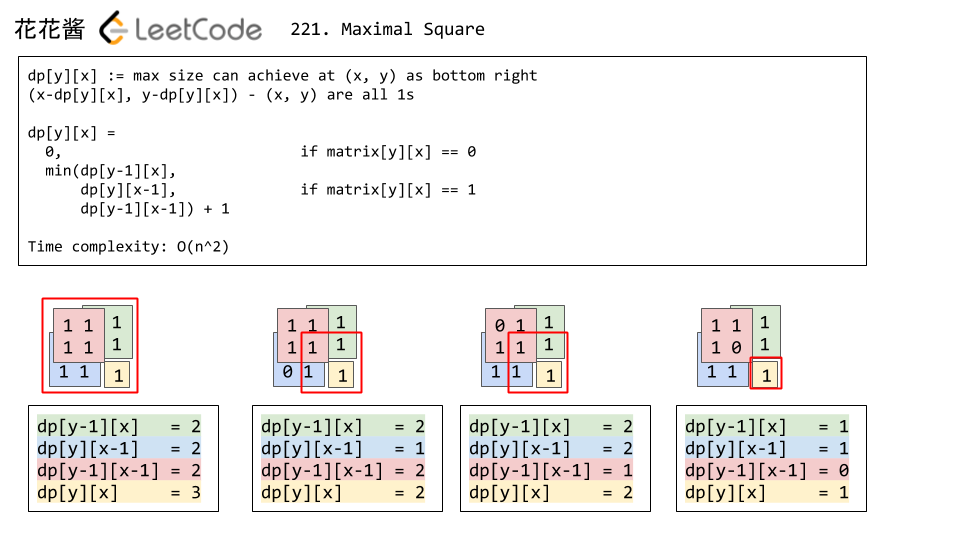# Posts tagged as “matrix”

Given a matrix A, return the transpose of A.

The transpose of a matrix is the matrix flipped over it’s main diagonal, switching the row and column indices of the matrix.

Example 1:

Input: [[1,2,3],[4,5,6],[7,8,9]]
Output: [[1,4,7],[2,5,8],[3,6,9]]


Example 2:

Input: [[1,2,3],[4,5,6]]
Output: [[1,4],[2,5],[3,6]]


Note:

1. 1 <= A.length <= 1000
2. 1 <= A.length <= 1000

# Solution: Brute Force

Time complexity: O(mn)

Space complexity: O(mn)

# Problem

Given an m * n matrix M initialized with all 0‘s and several update operations.

Operations are represented by a 2D array, and each operation is represented by an array with two positiveintegers a and b, which means M[i][j] should be added by one for all 0 <= i < a and 0 <= j < b.

You need to count and return the number of maximum integers in the matrix after performing all the operations.

Example 1:

Input:
m = 3, n = 3
operations = [[2,2],[3,3]]
Output: 4
Explanation:
Initially, M =
[[0, 0, 0],
[0, 0, 0],
[0, 0, 0]]

After performing [2,2], M =
[[1, 1, 0],
[1, 1, 0],
[0, 0, 0]]

After performing [3,3], M =
[[2, 2, 1],
[2, 2, 1],
[1, 1, 1]]

So the maximum integer in M is 2, and there are four of it in M. So return 4.


Note:

1. The range of m and n is [1,40000].
2. The range of a is [1,m], and the range of b is [1,n].
3. The range of operations size won’t exceed 10,000.

# Solution:

Time Complexity: O(n)

Space Complexity: O(1)

C++

A matrix is Toeplitz if every diagonal from top-left to bottom-right has the same element.

Now given an M x N matrix, return True if and only if the matrix is Toeplitz.
Example 1:

Example 2:

Note:

1. matrix will be a 2D array of integers.
2. matrix will have a number of rows and columns in range [1, 20].
3. matrix[i][j] will be integers in range [0, 99].

Idea:

Check m[i][j] with m[i-1][j-1]

Solution: Brute Force

Time complexity: O(n*m)

Space complexity: O(1)

C++

Java

Python3

Problem:

Given a 2D binary matrix filled with 0’s and 1’s, find the largest square containing only 1’s and return its area.

For example, given the following matrix:

1 0 1 0 0
1 0 1 1 1
1 1 1 1 1
1 0 0 1 0

Return 4.

Idea:

Dynamic programming

Solution 0: O(n^5)Solution 1: O(n^3)Solution 2: O(n^2)Solution 1:

Solution 2:

Mission News Theme by Compete Themes.